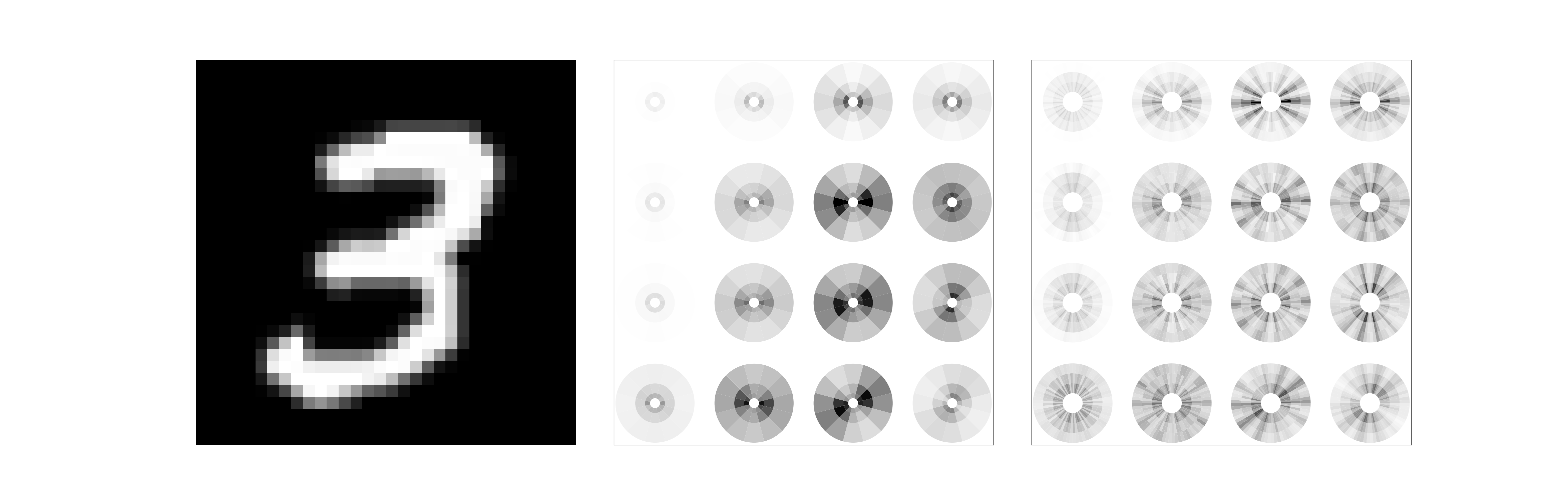# Scattering disk display¶

This script reproduces the display of scattering coefficients amplitude within a disk as described in “Invariant Scattering Convolution Networks” by J. Bruna and S. Mallat (2012) (https://arxiv.org/pdf/1203.1513.pdf).

Author: https://github.com/Jonas1312

Edited by: Edouard Oyallon and anakin-datawalker

import matplotlib as mpl
import matplotlib.cm as cm
import matplotlib.pyplot as plt
from matplotlib import gridspec
import numpy as np
from kymatio import Scattering2D
from PIL import Image
import os

img_name = os.path.join(os.getcwd(), "images/digit.png")


## Scattering computations¶

First, we read the input digit:

src_img = Image.open(img_name).convert('L').resize((32, 32))
src_img = np.array(src_img)
print("img shape: ", src_img.shape)


Out:

img shape:  (32, 32)


We compute a Scattering Transform withangles andscales.

Morlet waveletsare Hermitian, i.e:.

As a consequence, the modulus wavelet transform of a real signalcomputed with a Morlet waveletis invariant by a rotation ofof the wavelet. Indeed, since, we have.

Scattering coefficients of order:are thus invariant to a rotation ofof any wavelet. As a consequence, Kymatio computes scattering coefficients withwavelets whose orientation is uniformly sampled in an interval of length.

L = 6
J = 3
scattering = Scattering2D(J=J, shape=src_img.shape, L=L, max_order=2, frontend='numpy')


We now compute the scattering coefficients:

src_img_tensor = src_img.astype(np.float32) / 255.

scat_coeffs = scattering(src_img_tensor)
print("coeffs shape: ", scat_coeffs.shape)
# Invert colors
scat_coeffs= -scat_coeffs


Out:

coeffs shape:  (127, 4, 4)


There are 127 scattering coefficients, among which 1 is low-pass,are of first-order andare of second-order. Due to the subsampling by, the final spatial grid is of size. We now retrieve first-order and second-order coefficients for the display.

len_order_1 = J*L
scat_coeffs_order_1 = scat_coeffs[1:1+len_order_1, :, :]
norm_order_1 = mpl.colors.Normalize(scat_coeffs_order_1.min(), scat_coeffs_order_1.max(), clip=True)
mapper_order_1 = cm.ScalarMappable(norm=norm_order_1, cmap="gray")
# Mapper of coefficient amplitude to a grayscale color for visualisation.

len_order_2 = (J*(J-1)//2)*(L**2)
scat_coeffs_order_2 = scat_coeffs[1+len_order_1:, :, :]
norm_order_2 = mpl.colors.Normalize(scat_coeffs_order_2.min(), scat_coeffs_order_2.max(), clip=True)
mapper_order_2 = cm.ScalarMappable(norm=norm_order_2, cmap="gray")
# Mapper of coefficient amplitude to a grayscale color for visualisation.

# Retrieve spatial size
window_rows, window_columns = scat_coeffs.shape[1:]
print("nb of (order 1, order 2) coefficients: ", (len_order_1, len_order_2))


Out:

nb of (order 1, order 2) coefficients:  (18, 108)


## Figure reproduction¶

Now we can reproduce a figure that displays the amplitude of first-order and second-order scattering coefficients within a disk like in Bruna and Mallat’s paper.

For visualisation purposes, we display first-order scattering coefficientswithanglesspanningusing the central symmetry of those coefficients explained above. We similarly display second-order scattering coefficientswithanglesspanningbut keep onlyorientations for(and thus an interval of), so as not to overload the display.

Here, each scattering coefficient is represented on the polar plane within a quadrant indexed by a radius and an angle.

For first-order coefficients, the polar radius is inversely proportional to the scaleof the waveletwhile the angle corresponds to the orientation. The surface of each quadrant is also inversely proportional to the scale, which corresponds to the frequency bandwidth of the Fourier transform. First-order scattering quadrants can thus be indexed by.

For second-order coefficients, each first-order quadrant is equally divided along the radius axis by the number of increasing scalesand byalong the angle axis to produce a quadrant indexed by. It simply means in our case wherethat the first-order quadrant corresponding tois subdivided along its radius in 2 equal quadrants corresponding to, which are each further divided by thepossibleangles, and thatquadrants are only divided by, corresponding toand thepossible. Note that no second-order coefficients are thus associated toin this case whose quadrants are just left blank.

Observe how the amplitude of first-order coefficients is strongest along the direction of edges, and that they exhibit by construction a central symmetry.

# Define figure size and grid on which to plot input digit image, first-order and second-order scattering coefficients
fig = plt.figure(figsize=(47, 15))

gs = gridspec.GridSpec(1, 3, wspace=0.1)
gs_order_1 = gridspec.GridSpecFromSubplotSpec(window_rows, window_columns, subplot_spec=gs)
gs_order_2 = gridspec.GridSpecFromSubplotSpec(window_rows, window_columns, subplot_spec=gs)

# Start by plotting input digit image and invert colors
ax = plt.subplot(gs)
ax.set_xticks([])
ax.set_yticks([])
ax.imshow(255 - src_img, cmap='gray', interpolation='nearest', aspect='auto')

# Plot first-order scattering coefficients
ax = plt.subplot(gs)
ax.set_xticks([])
ax.set_yticks([])

l_offset = int(L - L / 2 - 1)  # follow same ordering as Kymatio for angles

for row in range(window_rows):
for column in range(window_columns):
ax.axis('off')
coefficients = scat_coeffs_order_1[:, row, column]
for j in range(J):
for l in range(L):
coeff = coefficients[l + j * L]
color = mapper_order_1.to_rgba(coeff)
angle = (l_offset - l) * np.pi / L
radius = 2 ** (-j - 1)
ax.bar(x=angle,
width=np.pi / L,
color=color)
ax.bar(x=angle + np.pi,
width=np.pi / L,
color=color)

# Plot second-order scattering coefficients
ax = plt.subplot(gs)
ax.set_xticks([])
ax.set_yticks([])

for row in range(window_rows):
for column in range(window_columns):
ax.axis('off')
coefficients = scat_coeffs_order_2[:, row, column]
for j1 in range(J - 1):
for j2 in range(j1 + 1, J):
for l1 in range(L):
for l2 in range(L):
coeff_index = l1 * L * (J - j1 - 1) + l2 + L * (j2 - j1 - 1) + (L ** 2) * \
(j1 * (J - 1) - j1 * (j1 - 1) // 2)
# indexing a bit complex which follows the order used by Kymatio to compute
# scattering coefficients
coeff = coefficients[coeff_index]
color = mapper_order_2.to_rgba(coeff)
# split along angles first-order quadrants in L quadrants, using same ordering
# as Kymatio (clockwise) and center (with the 0.5 offset)
angle = (l_offset - l1) * np.pi / L + (L // 2 - l2 - 0.5) * np.pi / (L ** 2)
radius = 2 ** (-j1 - 1)
# equal split along radius is performed through height variable
ax.bar(x=angle,
height=radius / 2 ** (J - 2 - j1),
width=np.pi / L ** 2,
bottom=radius + (radius / 2 ** (J - 2 - j1)) * (J - j2 - 1),
color=color)
ax.bar(x=angle + np.pi,
height=radius / 2 ** (J - 2 - j1),
width=np.pi / L ** 2,
bottom=radius + (radius / 2 ** (J - 2 - j1)) * (J - j2 - 1),
color=color)Total running time of the script: ( 0 minutes 11.079 seconds)

Gallery generated by Sphinx-Gallery